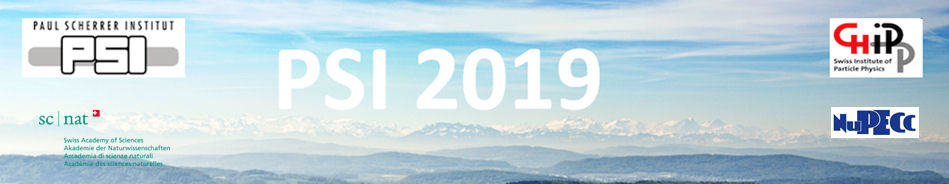#Physics of fundamental Symmetries and Interactions - PSI2019

Oct 20 – 25, 2019
PSI
Europe/Zurich timezone

## Probing neutrino nature and time reversal symmetry violation in elastic scattering of low energy neutrinos on polarized electrons in presence of nonstandard couplings

Oct 22, 2019, 4:45 PM
1m
WHGA/001 - Auditorium (PSI)

Poster

### Speaker

Dr Wieslaw Sobkow (Institute of Theoretical Physics, University of Wroclaw)

### Description

Possibility of using elastic scattering of low energy electron neutrinos ($\nu_e$s) on polarized electron target (PET) for testing time reversal symmetry violation (TRSV) and neutrino nature (NN) in leptonic interactions is considered. We analyze the two theoretically possible scenarios of physics beyond the Standard Model (SM) in which flavor-conserving (FC) standard and non-standard interactions of left chiral (LC) and FC exotic couplings of right chiral (RC) $\nu_e$s are admitted.
The first option assumes that the incoming $\nu_e$ beam consists only of LC $\nu_e$s detected by $V - A$ and $S, P, T$ interactions. It turns out that T-odd correlation built of the polarization of
the electron target, the incoming $\nu_e$ momentum and the outgoing electron momentum, and proportional to the interference between the S and T interactions in the cross section for the Dirac $\nu_e$s appears and results in a departure from the standard prediction for the azimuthal asymmetry of recoil electrons. The spectrum and polar distribution of recoil electrons are not sensitive to the T-odd correlation, but allow us to differentiate between Dirac and Majorana $\nu_e$s.
The second scenario is based on the assumption that the incoming $\nu_e$ beam is a superposition of LC states with RC ones. Consequently the transversal components of $\nu_e$ spin polarization (TCNSP) may appear and do not vanish in the limit of infinitesimally small $\nu_e$ mass.
LC $\nu_e$s interact mainly by the standard $V - A$ interaction, while RC ones are only detected by the exotic $V + A$ and $S_R, P_R, T_R$ interactions.
The differential cross section for Dirac $\nu_e$s contains the interference terms between standard $V-A$ and exotic $S_R, P_R, T_R$ couplings, while the differential cross section for the Majorana case does not include the interferences from V, T couplings. All the interferences are proportional to the various angular correlations (both T-even and T-odd) among the transversal
$\nu_e$ spin polarization, the polarization of the electron target, the incoming $\nu_e$ momentum and the outgoing electron momentum, and survive in the relativistic $\nu_e$ limit. In this way the azimuthal asymmetry, the electron spectrum and the polar angle distribution of the scattered electrons are sensitive to the TRSV and enable us to distinguish between Dirac and Majorana $\nu_e$s. The considerations are model-independent and carried out for the flavour (current) $\nu_e$s. To make such tests feasible, the intense (un)polarized artificial $\nu$ sources, PETs and the appropriate detector measuring both the polar and azimuthal angles of the outgoing electrons, and/or the recoil electrons energy with a high resolution have to be identified. This study is based on the published paper in the European Physical Journal C: W. Sobk\'ow, A. B\l{}aut, Eur. Phys. J. C {78}, 197 (2018), and the preprint arXiv:1812.09828.

### Primary author

Dr Wieslaw Sobkow (Institute of Theoretical Physics, University of Wroclaw)

### Co-author

Dr Arkadiusz Blaut (Institute of Theoretical Physics, University of Wroclaw)